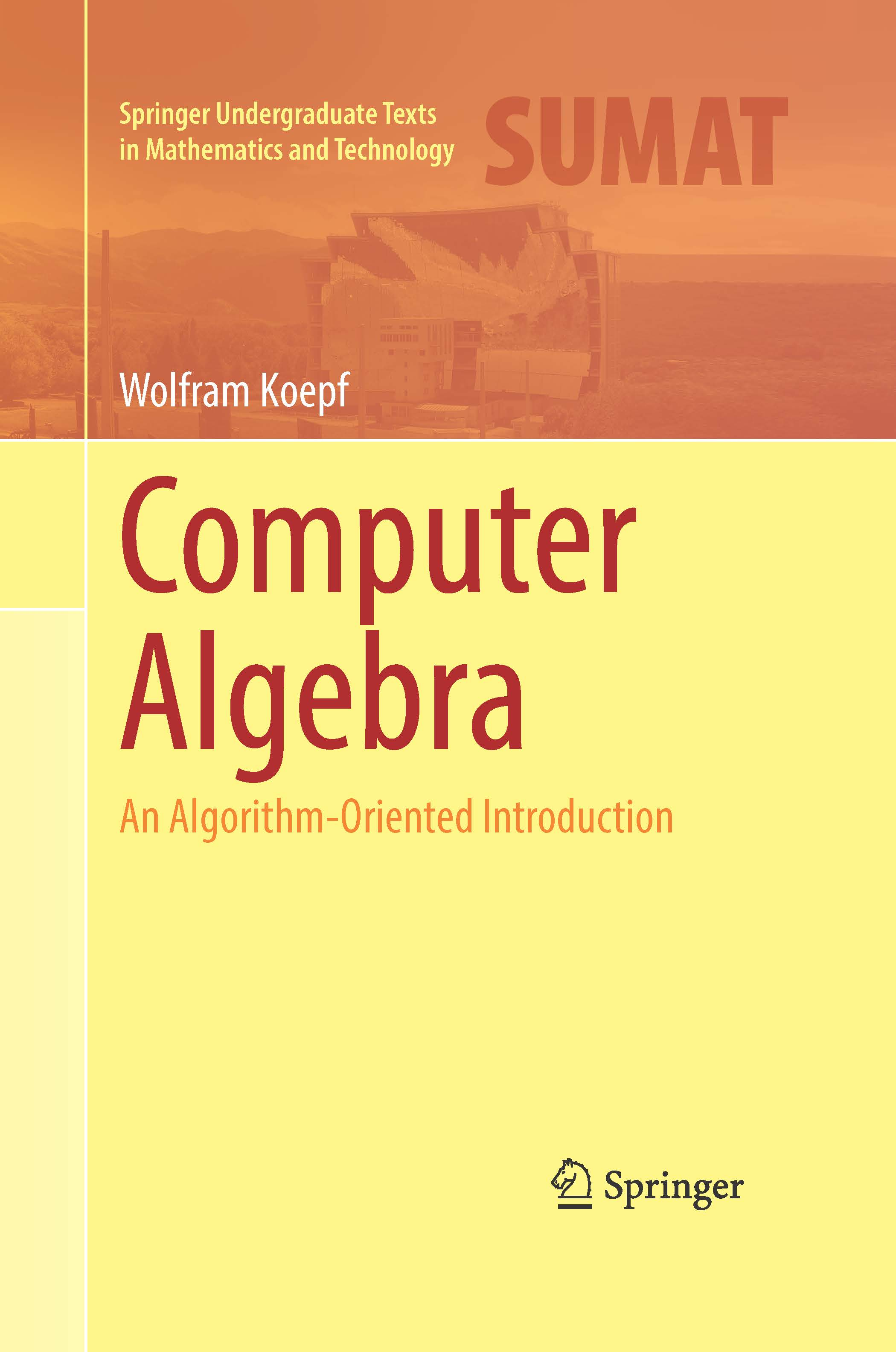Wolfram Koepf Computer Algebra - An Algorithm-Oriented Introduction Springer Undergraduate Texts in Mathematics and Technology (SUMAT), ISSN: 2021. XII, 384 pages. With 30 figures, numerous exercises and sample sessions Hardcover, € 57,19, ISBN: 978-3-030-78016-6

This textbook about computer algebra gives an introduction to this modern field of Mathematics. The contents of the first eight chapters seem to be an indispensable foundation of computer algebra and therefore form an introductory course. Chapters 9 to 12 build the basis of a more advanced lecture on computer algebra and are influenced by the author's own research interests.

Instead of describing algorithms—as in most other books—by so-called pseudo code, all algorithms considered in this book are implemented and presented as executable programs in the general-purpose computer algebra system Mathematica. Nevertheless, lecturers are absolutely not bound to this system, since the book’s definitions and theorems are completely independent of a chosen computer algebra system. Consequently, all computer algebra sessions can be downloaded as worksheets in the three systems Mathematica, Maple and Maxima from this internet page. Therefore every lecturer can select his or her own favorite system. In particular, Maxima is freely available and was used by the author when teaching the same course several times in Africa at AIMS Cameroon.

By using real implementations instead of pseudo-code, the considered algorithms are immediately applicable and verifiable. Knowledge from higher algebra is not required, nevertheless all proofs are given. Since the book is rather elementary and contains a detailed index, it can also be used as a reference manual on algorithms of computer algebra.

The author lectured and did research in the field of computer algebra in the last three decades and was the chairman of the German Fachgruppe Computeralgebra from 2002-2011.

All sessions as zip-archivs: Mathematica, Maple, Maxima. I am indebted to Dr. Bertrand Teguia Tabuguia who created the Maple and Maxima worksheets.

Preface
Chapter 1: Introduction to Computer Algebra . Capabilities of Computer Algebra Systems
Chapter 2: Programming in Computer Algebra Systems . Internal Representation of Expressions . Pattern Matching . Control Structures . Recursion and Iteration . Remember Programming . Divide-and-Conquer Programming . Programming through Pattern Matching
Chapter 3: Number Systems and Integer Arithmetic . Number Systems . Integer Arithmetic: Addition and Multiplication  . Integer Arithmetic: Division with Remainder . The Extended Euclidean Algorithm . Unique Factorization . Rational Arithmetic
Chapter 4: Modular Arithmetic . Residue Class Rings . Modulare Square Roots . Chinese Remainder Theorem . Fermat’s Little Theorem . Modular Logarithms . Pseudoprimes
Chapter 5: Coding Theory and Cryptography . Basic Concepts of Coding Theory . Prefix Codes . Check Digit Systems . Error Correcting Codes . Asymmetric Ciphers
Chapter 6: Polynomial Arithmetic . Polynomial Rings . Multiplication: The Karatsuba Algorithm . Fast Multiplication with FFT . Division with Remainder . Polynomial Interpolation . The Extended Euclidean Algorithm . Unique Factorization . Squarefree Factorization . Rational Functions
Chapter 7: Algebraic Numbers . Polynomial Quotient Rings . Chinese Remainder Theorem . Algebraic Numbers . Finite Fields . Resultants . Polynomial Systems of Equations
Chapter 8: Factorization in Polynomial Rings . Preliminary Considerations . Efficient Factorization in Zp[x] . Squarefree Factorization of Polynomials over Finite Fields . Efficient Factorization in Q[x] . Hensel Lifting . Multivariate Factorization
Chapter 9: Simplification and Normal Forms . Normal Forms and Canonical Forms . Normal Forms and Canonical Forms for Polynomials . Normal Forms for Rational Functions . Normal Forms for Trigonometric Polynomials
Chapter 10: Power Series . Formal Power Series . Taylor Polynomials . Computation of Formal Power Series . Holonomic Differential Equations . Holonomic Recurrence Equations . Hypergeometric Functions . Efficient Computation of Taylor Polynomials of Holonomic Functions . Algebraic Functions . Implicit Functions
Chapter 11: Algorithmic Summation . Definite Summation . Difference Calculus . Indefinite Summation . Indefinite Summation of Hypergeometric Terms . Definite Summation of Hypergeometric Terms
Chapter 12: Algorithmic Integration . The Bernoulli Algorithm for Rational Functions . Algebraic Prerequisites . Rational Part . Logarithmic Case
References . List of Symbols . Mathematica List of Keywords . Index

Wolfram Koepf
July 05, 2021